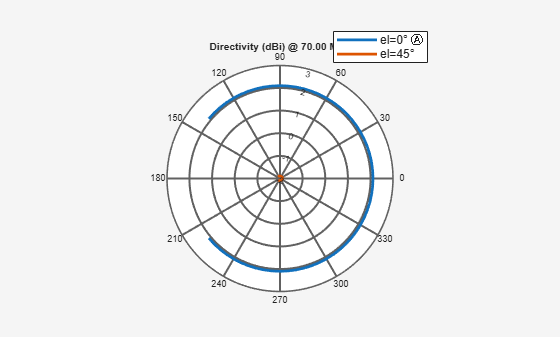# patternAzimuth

Azimuth plane radiation pattern of antenna or array

## Syntax

``patternAzimuth(object,frequency,elevation)``
``patternAzimuth(object,frequency,elevation,Azimuth=Value)``
``directivity = patternAzimuth(object,frequency,elevation)``
``directivity = patternAzimuth(object,frequency,elevation,Azimuth=Value)``

## Description

example

````patternAzimuth(object,frequency,elevation)` plots the 2-D radiation pattern of the antenna or array object in the azimuth plane over a specified frequency. Elevation values default to zero if not specified.```

example

````patternAzimuth(object,frequency,elevation,Azimuth=Value)` uses the specified range of azimuth angles to plot the 2-D radiation pattern of the antenna or array in the azimuth plane over a specified frequency. Elevation values default to zero if not specified. Azimuth values default to `[-180:1:180]` if not specified.```
````directivity = patternAzimuth(object,frequency,elevation)` returns the directivity of the antenna or array object over a specified frequency. Elevation values defaults to zero if not specified.```
````directivity = patternAzimuth(object,frequency,elevation,Azimuth=Value)` uses the specified range of azimuth angles to calculate the directivity of the antenna or array object over a specified frequency. Elevation values default to zero if not specified. Azimuth values default to `[-180:1:180]` if not specified.```

## Examples

collapse all

Calculate and plot the azimuth radiation pattern of the helix antenna at 2 GHz.

```h = helix; patternAzimuth(h,2e9);```Calculate and plot the azimuth radiation pattern of the dipole antenna at 70 MHz at elevation values of 0 and 45.

``` d = dipole; patternAzimuth(d,70e6,[0 45],Azimuth=-140:5:140);```## Input Arguments

collapse all

Antenna or array from catalog, custom antenna or array, or installed antenna on a platform to calculate/plot the azimuth plane directivity pattern, specified as an antenna or array object.

Example: `dipole`

Example: `rectangularArray`

Frequency to calculate/plot azimuth directivity pattern, specified as a scalar in Hz.

Example: 70e6

Data Types: `double`

Elevation angle values, specified as a vector in degrees.

Example: `[0 45]`

Data Types: `double`

Range of azimuth angles to calculate the directivity of antenna or array, specified as a vector in degrees.

Example: `Azimuth=2:2:340`

Data Types: `double`

## Output Arguments

collapse all

Antenna or array directivity, returned as a matrix in `dBi`. The matrix size is the product of number of elevation values and number of azimuth values.

## Version History

Introduced in R2015a